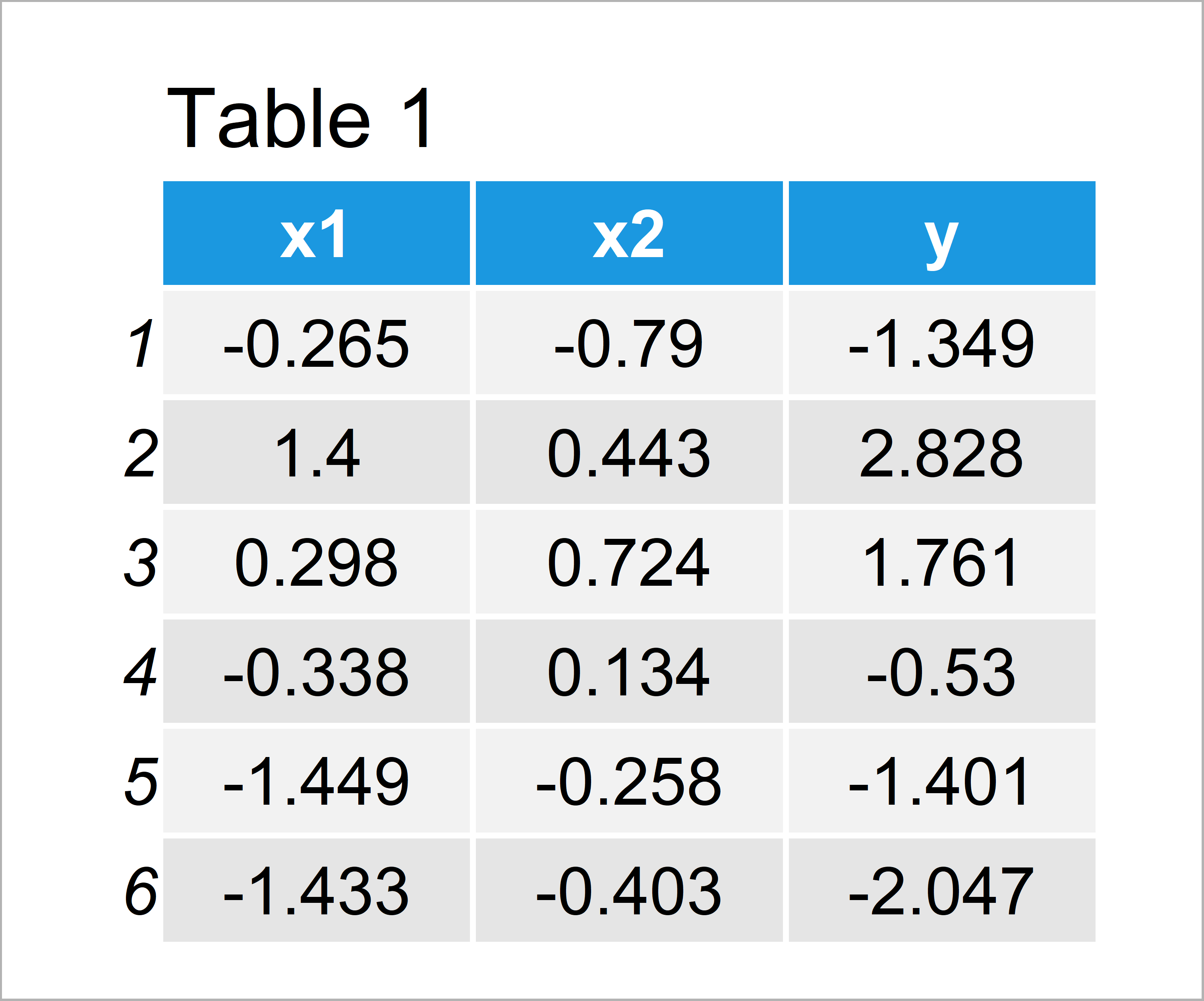# Remove Intercept from Regression Model in R (2 Examples)

In this tutorial you’ll learn how to estimate a linear regression model without intercept in the R programming language.

Let’s get started!

## Example Data

The following data is used as basement for this R tutorial:

```set.seed(23985742)                               # Create example data
x1 <- rnorm(100)
x2 <- rnorm(100) + 0.2 * x1
y <- rnorm(100) + x1 + x2
data <- data.frame(x1, x2, y)Table 1 shows that our exemplifying data has 100 rows and three columns. The variable x1 and x2 will be used as predictors and the variable y will be our outcome variable.

## Example 1: Estimate Linear Regression Model with Intercept

In Example 1, I’ll explain how to estimate a linear regression model with default specification, i.e. including an intercept.

In the following R code, we use the lm function to estimate a linear regression model and the summary function to create an output showing descriptive statistics of our model:

```mod_intercept |t|)
# (Intercept) -0.03848    0.09480  -0.406    0.686
# x1           0.90243    0.10169   8.874 3.61e-14 ***
# x2           0.87321    0.09835   8.879 3.53e-14 ***
# ---
# Signif. codes:  0 ‘***’ 0.001 ‘**’ 0.01 ‘*’ 0.05 ‘.’ 0.1 ‘ ’ 1
#
# Residual standard error: 0.9415 on 97 degrees of freedom
# Multiple R-squared:  0.6761,	Adjusted R-squared:  0.6695
# F-statistic: 101.3 on 2 and 97 DF,  p-value: &lt; 2.2e-16
#```

As you can see in the previous output of the RStudio console, our regression model shows estimates for the two independent variables x1 and x2 as well as for the intercept.

Do you want to get rid of the intercept? Keep on reading…

## Example 2: Remove Intercept from Linear Regression Model

Example 2 illustrates how to delete the intercept from our regression output.

Please note: This tutorial does not discuss whether it is good or bad to remove the intercept from a regression model. Have a look here for a detailed discussion on this topic.

However, in case you have decided to remove the intercept from a regression model, then you might specify that by adding “0 +” in front of the model formula.

Have a look at the following R code and its output:

```mod_no_intercept |t|)
# x1  0.90399    0.10118   8.934 2.48e-14 ***
# x2  0.87717    0.09744   9.002 1.77e-14 ***
# ---
# Signif. codes:  0 ‘***’ 0.001 ‘**’ 0.01 ‘*’ 0.05 ‘.’ 0.1 ‘ ’ 1
#
# Residual standard error: 0.9375 on 98 degrees of freedom
# Multiple R-squared:  0.6798,	Adjusted R-squared:  0.6733
# F-statistic:   104 on 2 and 98 DF,  p-value: &lt; 2.2e-16
#```

As you can see, the previous descriptive statistics do not show estimates for the intercept. You can also see that the estimates for x1 and x2 have changed due to the removal of the intercept.

## Video, Further Resources & Summary

Some time ago I have published a video on my YouTube channel, which shows the R programming codes of this article. You can find the video below.

Please accept YouTube cookies to play this video. By accepting you will be accessing content from YouTube, a service provided by an external third party.If you accept this notice, your choice will be saved and the page will refresh.

In addition, you might want to have a look at the related tutorials on my homepage. You can find some posts on related topics such as extracting data and regression models here:

To summarize: In this R programming post you have learned how to delete the intercept from a multiple regression. If you have additional questions, let me know in the comments. Besides that, don’t forget to subscribe to my email newsletter in order to receive regular updates on new posts.

Subscribe to the Statistics Globe Newsletter